﻿ A New Equivalent Theory with Special Relativity Theory, Particle Wave in 5-dimensional Space-time

### A New Equivalent Theory with Special Relativity Theory, Particle Wave in 5-dimensional Space-time

Xiao Lin Li

International Journal of Physics

## A New Equivalent Theory with Special Relativity Theory, Particle Wave in 5-dimensional Space-time

Chongqing, China

### Abstract

There exist a new physics model. Real physical world is 5-dimensional space-time. Human world is 4-dimensional space-time. Human world just is the projection of real physics world. Particle wave is present in 5-dimensional space-time. So we can derive out Mass-energy equation. So we can derive out all results of Special Relativity Theory. In 5-dimensional space-time, speed of all particles is the light speed c. It is reason that the light speed c is very special. Coordinates transformation in 5-dimensional space-time, can derive out Lorentz transformation. The new physics model theory is a equivalent theory with Special Relativity Theory. In 5-dimensional space-time, there only exist space expansion or space contraction. In 5-dimensional space-time, there not exist time expansion or time contraction, time is absolute. In 5-dimensional space-time, particle rest mass only is particle movement portions at 4th dimensional space. The new 5-dimensional space-time theory, is consistent with space-time system of Special Relativity Theory, and it can transition to space-time system in Lorentz symmetry breaking smoonthly. The new 5-dimensional space-time theory has more wide range adaptation than Special Relativity Theory.

• Xiao Lin Li. A New Equivalent Theory with Special Relativity Theory, Particle Wave in 5-dimensional Space-time. International Journal of Physics. Vol. 4, No. 5, 2016, pp 123-129. http://pubs.sciepub.com/ijp/4/5/2
• Li, Xiao Lin. "A New Equivalent Theory with Special Relativity Theory, Particle Wave in 5-dimensional Space-time." International Journal of Physics 4.5 (2016): 123-129.
• Li, X. L. (2016). A New Equivalent Theory with Special Relativity Theory, Particle Wave in 5-dimensional Space-time. International Journal of Physics, 4(5), 123-129.
• Li, Xiao Lin. "A New Equivalent Theory with Special Relativity Theory, Particle Wave in 5-dimensional Space-time." International Journal of Physics 4, no. 5 (2016): 123-129.

 Import into BibTeX Import into EndNote Import into RefMan Import into RefWorks

### 1. Introduction

We know. There exist de Broglie wave,or particle wave,in Quantum Mechanics. To particle wave,there exist two formula, de Broglie formula.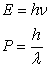The particle wave concept, is a basic concept in Quantum Mechanics. The de Broglie formula is a basic principle in Quantum Mechanics. De Broglie formula can not be derive out from Quantum Mechanics. De Broglie formula can not be derive out from Special Relativity Theory also. It is a independent and basic principle in current physics theory. It is correct or wrong, only the physical experiment can do the judgement.

In physical history, many scientists, include de Broglie, want to find the relationship between de Broglie formula and Special Relativity Theory. But not success.

We know. Special Relativity Theory is rely on light speed c strictly. In Special Relativity Theory, space and time is symmetry strictly. Once the light speed c is not invariant, Special Relativity Theory will be no longer valid. In Quantum Field Mechanics, many scientists take out the possibility of Lorentz symmetry breaking. So, once the Lorentz symmetry breaking evidence has been found by experiment, Special Relativity Theory will be incorrect, so space and time is not symmetry again, new space-time theory will be necessary. So we need to try to study any new model about space-time.

After continuous exploration, author find a very interesting result. If we extend the particle wave and the de Broglie formula to 5-dimensional space-time, we can simply derive out all results of Special Relativity Theory. Author have found the relationship between de Broglie wave and Special Relativity Theory successfully. By this way, author put forward a new 5-dimensional space-time theory. The new theory is a equivalent theory with Special Relativity Theory.

At next chapter, author will explain this new theory in detail.

### 2. Derive out Energy-mass Equation from de Broglie Formula

In the quantum mechanics, the particle wave group velocity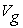is equal with particle velocity V. That is=V.

The phase velocity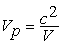So we obtain,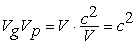So we obtain a equation,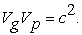(2.1)

The equation (2.1) is derived. But we think from the other side. We suppose this equation is exist at first, not be derived out. The physics source will be explained at the next chapter. Start from this equation, do some logical reasoning, we can obtain very meaningful results.

From de.Broglie equation, we obtain a equation,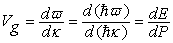(2.2)

Please pay attention to the equation,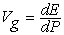.

From de.Broglie equation, we can derive out, the phase velocity,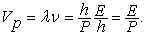(2.3)

Take (2.3) and (2.2) into (2.1),we obtain,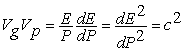So we obtain a equation,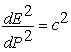(2.4)

So we obtain,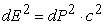.

So, we obtain,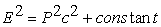(2.5)

This equation is so familiar. It’s more like energy-momentum equation in Special Relativity Theory. The constant is wait solving. Now we start to solve the constant value.

The group velocityis equal with particle velocity V. That is=V.

Exist equation,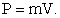(2.6)

So,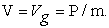(2.7)

Take (2.3) and (2.7) into (2.1), get,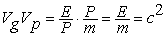So obtain,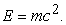(2.8)

Equation (2.8) is the energy-mass equation in Special Relativity Theory.

Take (2.8) into (2.5), obtain,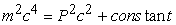So we can set the constant is value when P=0.

We set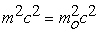when P=0.So,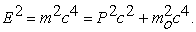(2.9)

This is the energy-mass equation in Special Relativity Theory.

Derive out equation (2.9), so we can derive out all results in Special Relativity Theory. From equation (2.9), we can derive out this result that the light speed c is the maximum speed.

Summarize the reasoning steps above again. We suppose exist the equationat first. Then we use de.Broglie formula. Then we obtain equation. Then use the equation P=mV. So we can derive out equation (2.9), and can energy-mass equation.

We can discover, the equation,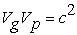(), is so much important. But why it is exist? It will be explained at the next chapter.

In fact, the equation, P=mV. It’s usage is that take mass concept into this theory. This mass is momentum mass. Momentum mass have another name, inertial mass. This mass is not gravitational mass.

From the reasoning steps above, we can see this. Before use equation P=mV, from (2.1) to (2.5), reasoning don’t have relationship with mass concept.

Use equation (2.6), we take mass concept into this theory. Then we can derive out equation (2.9). (2.9) is the energy-mass equation.

Why=V? In Classical mechanics, dP=Fdt, dE=Fds.So, dE/dP=Fds/Fdt=ds/dt=V. From de.Broglie equation, can obtain (2.2), Vg=dE/dP. So we obtain=V.

Reasoning steps above seems only formal changes, it is not substantive changes. But, if our thinking is no longer been limited by Special Relativity Theory. Then we can get new thinking.

In fact, the, only is a special case. The wide case is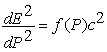(2.10)

When f(P) =constant, we can get, so we can derice out energy-mass equation, so we can derive out all results in Special Relativity Theory. This means that the new thinking theory is equivalent with Special Relativity Theory.

If we change f(P). f(P) is other form. This will lead to many Lorentz-Violating Extension case. To f(P),there exist many other form. The (2.10) formula is a theory pattern. We can consider that Special Relativity Theory is one type of this pattern. About this theory pattern, it is a topic worthy of further study.

### 3. New Physics Model, Particle Wave in 5-dimensional Space-time

In above chapter, this equation,, is a hypothesis. So, what is its physical meaning? Thoughts two years, author finally found its physics significance. This equation’s physical model, will bring a new view of physics.

This equation means that the particle wave is present in 5-dimensional space-time. The physical process of human perception, just is the spatio-temporal projection on the 4-dimensional space-time. Real physical process happen in 5-dimensional space-time.

The physical world of human perception, is in a 4-dimensional space-time. One dimension is time. Three dimension is space. Now we assume that a real physical world is in 5-dimensional space-time. There exist 5 dimensions. One dimension is time. Four dimension is space. Real physical world is in 4-dimensional space. Humans live in 3-dimensional space. The physical process of human perception, is only the projection on the 3-dimensional space.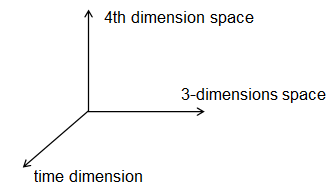The new 4th dimension space, and 3-dimensional space, forming orthogonal relationships. Total of 4-dimensional space is a Hilbert space. This like 3-dimensional space also. The vector operations in 4-dimensional space, follow the standard vector arithmetic rules also.

Because there add a new dimension. And velocity and momentum is vectors, not scalars. In the new 4-dimensional space, particle movement and particle wave movement, has very different property, compare with movement property in 3-dimensional space.

However, mass, energy, wavelength, frequency. To these physical quantity, because it is a scalar, then there not have difference.

Now, we assume that, in new 4-dimensional,the wave particle duality still holds, De.Broglie equation still holds.(3.1)

Please notice that the frequency and wavelength, are in 4-dimensional space, not just in 3-dimensional space. Energy and momentum are in 4-dimensional space, not just in 3-dimensional space. The velocity of the particle, particle-wave phase velocity and group velocity, is a 4-dimensional vector, not a 3-dimensional vector.

To energy and frequency, in 4-dimensional space, and in 3-dimensional space, its value are same. Because it is a scalar.

To momentum and velocity, in 4-dimensional space, and in 3-dimensional space, it is not the same. Because it is a vector.

To wavelength, it is a length quantity, is closely related with dimensions. It is not a scalar or vector. In 4-dimensional space, and in 3-dimensional space, it is also not the same.

In the following statements, please reader distinguish each physical quantity carefully. It is in 4-dimensional space, or it is in 3-dimensional space.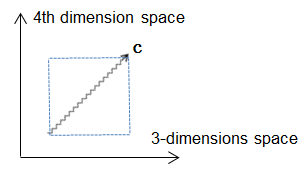Now, we assume that, in the new 4-dimensional space, particle-wave phase velocity and the Group velocity is the same, and its numeric value is the speed of light c. And, in the new 4-dimensional space, all particles, all particle wave, movement speed is light speed c. In 4-dimensional space, there not exist static particle, and not exist static particle wave. As long as the particle exist, it is in movement at the speed of light c necessarily, inevitably accompanied by the particle-wave. And, in the new 4-dimensional space, the Group velocity of the particle-wave, and particle velocity of the particle-wave , have same value,=V.

So, obtain,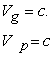(3.2)

So, we obtain this equation,(3.3)

Please notice that distinction, the phase velocity and the Group velocity, is in new 4-dimensional space, is not only in 3-dimensional space.

We can see that, the vast majority of cases, to the Group velocity of the particle-wave, its projection speed in 3-dimensional space, is no more than a numerical vector which in the 4-dimensional space. So, in 3-dimensional space, particle velocity, which is the Group velocity of projection speed, do not exceed the speed of light c. Only in extreme cases, the particle velocity completely only have the component in 3-dimensional space. In the 4th dimension, its velocity component is zero. The projection speed in 3-dimensional space, is entirely the vector itself values. The particle speed is the speed of light c in 3-dimensional space. Photon are examples of this status.

Then, there exist another special case. Particle-wave group velocities, complete only have the component in 4th dimension. In 3-dimensional space, its projection components, completely to zero. This is a what? In fact, in this case, is the static object in 3-dimensional space.

In 3-dimensional space, the particle is static, not move, it was just an illusion. In new 4-dimensional space, the particle movement is at the speed of light c. Therefore, the particle has energy. Energy is a scalar, not a vector, its physical effects in each dimension will show effect. This is the physical source that particle have rest mass in the 3-dimensional space. But the particle velocity and momentum is a vector, orthogonal component is zero in 3-dimensional space, the projection is zero, so it will not show effects. This is a proper physical explanation about particle rest mass. Why particle-wave have frequency and wavelength when particle is static in 3-dimensional space? This is its physical explanation.

To phase velocity, because it involves the wavelength, than the Group velocity, is more complex. Obviously, lengths is closely associated with dimensions. The length in 4-dimensional space, the projection length in 3-dimensional space, they have what kind of relationship, it is not sure. Wavelength in 4-dimensional space, and projection of the wavelength in 3-dimensional space, is clearly not a simple vector orthogonal relationships. The relationships is not clear also. It is still a problem that requires careful study.

Exist a equation:(3.4)

Its usage is that take mass concept into theory. This mass is momentum mass. Momentum mass have another name, inertial mass. This mass is not gravitational mass. But please notice that the momentum here, is the momentum in 4-dimensional space. Here the velocity V, is in 4-dimensional space also. But the mass quality is a scalar, so in 4-dimensional space, and in 3-dimensional space, is same, have same numeric value.

Because in 4-dimensional space, the particle speed V is the speed of light c. And=V. So, (3.4) equation is equivalent to this: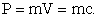(3.5)

But it is correct only in 4-dimensional space. In 3-dimensional space, it is incorrect.

The momentum in 4-dimensional space, have an orthogonal component in 3-dimensional space. Mark the orthogonal component in 3-dimensional space with lowercase p. There exist p=mv. Here, p is the 3-dimensional momentum, v is a 3-dimensional velocity. m is a scalar.

In 4-dimensional space, from (3.1) and (3.2), can obtain: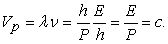And (3.5), so obtain: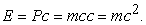(3.6)

We assume, in 5-dimensional space-time (4-dimensional space,1-dimensional time),have V=Vg also. So obtain,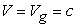(3.7)

To the momentum P in 4-dimensional space, have an orthogonal component in 3-dimensional space (mark with P1), and have an orthogonal component in new 4th dimension(mark with P2). There exist,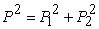. And (3.6), so obtain: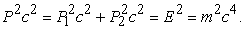When particle is static in 3-dimensional space, P1=0, P=P2. Named this mass with rest mass. Mark this mass with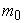.So obtain: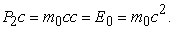(3.8)

So obtain: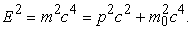(3.9)

Here, p=P1, is the momentum component in 3-dimensional space. Therefore, the energy-mass equation in Special Relativity Theory, is a simple result of this new physical view. In the new view, the result of energy-mass equation is very direct, not exist complex reasoning process in Special Relativity Theory.

Why the mass-energy equation shows a strict right angle triangle relationship, which is a very strange phenomenon. This is many people's doubts. On this issue, the new physical view, can give a very direct answer. Indeed, mass-energy equation, is a vector orthogonal relationship performance.

As can be seen, the momentum of the particle in the 4th dimension component values, is a variable that will always remain the same. This is the particle rest mass in 3-dimensional space. Why it is not chang? It need further study.

In 4-dimensional space, there is no static particle. But judging from the macroscopic physical, in 3-dimensional space, there exist static object. Why? It need further study.

From the above discussion, we can see that particle mass is a human artificial concept. Mass concept is taked into physics is by a equation, P=mV. Mass seems to not have real physical meaning. Energy, momentum, speed, frequency, wavelength, these concepts, have a real physical meaning. Therefore, the real physical meaning of mass quality, need further study.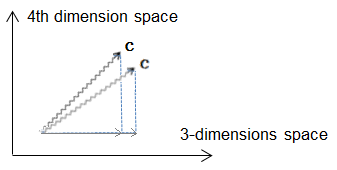From the diagram above, we can see also, the energy-momentum of the particle increases, showed speed in 3-dimensional space projection component mounting. In 4-dimensional space, the real is the rotation of the particle velocity vector in the 4th dimension. Greater energy-momentum particle movement and 3-dimensional orthogonal angle is smaller, and the 4th dimension orthogonal angles greater. There only exist rotation of velocity vector. The value of velocity vector is invariant.

The photon is the most exceptional circumstances, only have motion components in 3-dimensional space completely, not have moving components in the 4th dimension, so the photon rest mass is zero, so the photon speed is equal to the light speed c in 3-dimensional space.

In 5-dimensional space-time, all particles, including photons, its movement speed is the speed of light, is c. This is the essence of Lorentz symmetry. Photons are no longer in a special place in physics. Ignore particle charge, spin, etc property. Only study on kinematics, not inspect on kinetics, all particles is same. Particle rest mass in the 3-dimensional space is different, only because particle’s moving components in the 4th dimension space is different. This means that, there exist a new particle symmetry in 5-dimensional space-time.

In this new model, the 1-dimensional time, and the 4-dimensional space, is not symmetry again. The time dimension will return back to absolute time dimension in Newtonian mechanics. The time dimension in every coordinates is synchronous. But, in this new model, the space dimensions, is not same with space dimension in Newtonian mechanics. In this new model, space dimensions will be expanding or contracted when coordinates transformation. This will be discussed in detail at next chapter.

This new physics model, have a equivalent relation with Special Relativity Theory. Coordinates transformation in Special Relativity Theory is a physics equivalent effect in the new 5-dimensional space time theory. This will be discussed in detail at next chapter.

### 4. Coordinates Transformation in 5-dimensional Space-time

In 5-dimensional space-time model, particle wave speed value is invariant. This speed value is light speed c always. So, coordinates transformation of different observer, is only a rotate transform of the velocity vector in 4-dimensional sapce. The value of velocity vector is invariant. The transformation effect is that change direction of motion. This effect is that rotate the velocity vector only, not change the value of velocity vector.

Imitate Special Relativity Theory, we take next derivation.

In A coordinates, particle coordinates values is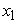,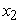,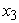, and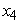. Start from coordinates origin.There exist this formula.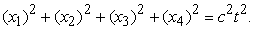(4.1)

In B coordinates, particle coordinates values is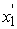,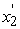,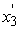, and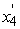. Start from coordinates origin also.There exist this formula.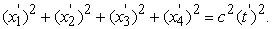(4.2)

In two coordinates, the time dimension is absolute time. Two time value is equal. So exist.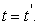(4.3)

Imitate Special Relativity Theory, change style, can get two formula.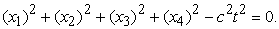(4.4)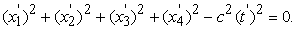(4.5)

So can get.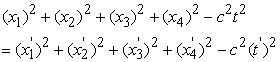(4.6)

The (4.6) formula, is coordinates transformation formula in 5-dimensional space-time model.

Because the time dimension is absolute, its same in all coordinates, so exist (4.3) formula. So can simplify (4.6), get (4.7).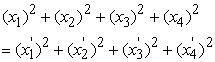(4.7)

(4.7) is a rotate transform of the velocity vector in 4-dimensional space. The value of velocity vector is invariant. The transformation effect is that change direction of motion. This effect is that rotate the velocity vector only, not change the value of velocity vector. This result is very obvious. In different coordinates, will observing space expansion or space contraction.

From (4.6), we can derive out coordinates transformation formula of Special Relativity Theory.

Formula (4.6) is coordinates transformation formula in 5-dimensional space-time. But human live in 4-dimensional space-time. Human only can sense the 3-dimensional space. Human can not sense the 4th dimension space. So, to human, in (4.6) formula, there don’t existand. But human can’t change the fact physics process. The (4.6) formula is the fact physics process. How to solve this problem? Key is the time dimension.

Human can’t sense the 4th dimension space . So human consider that not existand. So human removeandfrom (4.6) formula. So human get (4.8) formula.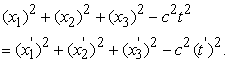(4.8)

But in (4.8) formula, the time dimension,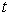and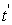, is not equal again.

(4.8) formula, is the coordinates transformation formula of Special Relativity Theory.

To human, the 4th dimension space expansion or contraction, is replaced by time dimension expansion or contraction. This is the mystery of Special Relativity Theory. Human observing time dimension expansion or contraction, this is only a observation effects. This is not the fact physics process. But human can’t distinguish the difference between observation effects and fact physics process, because human can’t sense the 4th dimension space. Human consider that time dimension expansion or contraction is fact physics truth. Human consider that space and time is symmetry.

So, from above description, we can get this derivation. The coordinates transformation in 5-dimensional space-time contains the coordinates transformation of Special Relativity Theory.

If there exist another intelligent biology in universe, which can sense the 4th dimension space. To this intelligent biology, they observing physics process, they will take the (4.6) formula, not take the (4.8) formula.

This likes the observation question in quantum mechanics. Human observing physics process, is equal to the fact physics process? To space-time, human observing space-time, is equal to the fact physics space-time? Human observing results, 100% is equal to fact physics process?

Human can’t distinguish the difference between Special Relativity Theory and 5-dimensional space-time theory. So, to physics, what significance can take out by the new 5-dimensional space-time model? The new 5-dimensional space-time model is only a duplicate theory with Special Relativity Theory? No. the significance taked out by the new 5-dimensional space-time theory is that transform to Lorentz symmetry breaking smoothly.

The new 5-dimensional space-time model, is integration between particle wave and space-time theory. The new model can derive out results of Special Relativity Theory also. To the new model, the most important is that time and space is separate. The new model contains that space and time symmetry in 4-dimensional space-time. And the new model contains that space and time is not symmetry 5-dimensional space-time also. The new model not only is consistent with space-time system of Special Relativity Theory, but also can transition to space-time system in Lorentz symmetry breaking smoonthly. The new model has more wide range adaptation than Special Relativity Theory.

Special Relativity Theory is rely on light speed c strictly. In Special Relativity Theory, space and time is symmetry strictly. Once the Lorentz symmetry breaking evidence has been found by experiment, Special Relativity Theory will be incorrect, so space and time is not symmetry again. New space-time theory will be necessary. In the new 5-dimensional space-time theory, space and time is not symmetry. So the new model can transition to Lorentz symmetry breaking smoonthly.

The new 5-dimensional space-time theory can explain physics process when space and time is symmetry. The new 5-dimensional space-time theory can explain physics process when space and time is not symmetry also. This is value of the new model.

The new 5-dimensional space-time theory can explain three physics process that Special Relativity Theory can not explain.

1. The source of particle rest mass. In current theory, particle rest mass is a property by human set and measuring. But human can’t explain the source of rest mass. In new 5-dimensional space-time theory, particle rest mass just is particle movement portions at 4th dimensional space. When observing in 5-dimensional space-time, particle don’t have rest mass. All particles, include photon, have same movement property. Obviously, in 5-dimensional space-time model, particle will have a new symmetry. This new symmetry will take out what influence, this topic is worth studying.

2. In Quantum Mechanics, when particle is static, particle have wave movement also. At this time, particle wave has limited frequency, but has unlimited wavelength. This is a confusing question. This is not easy to understand. In fact, this is a theory weakness. In new 5-dimensional space-time model, this question has been explained reasonably. When particle is static in 4-dimensional space-time system, but particle is moving in 5-dimensional space-time system. The particle wave is in 5-dimensional space-time. So particle has limited frequency and limited wavelength. The unlimited wavelength is a semblance, not the fact.

3. Space is relative, time is absolute. This can bring logical consistency of theory. Time expansion or contraction in Special Relativity Theory, it’s truth is frequency and wavelength change of particle wave. This only is transform effect, not the physics fact. In Quantum Mechanics, all physics process is particle wave process. When coordinates transformation, all particle wave frequency and wavelength will change. So all physics process frequency will change. This is truth of Time expansion or contraction in Special Relativity Theory. But humen live in 4-dimensional space-time system, can’t distinguish the difference between time change and frequency change. Human measuring time, must use equipment. Measuring time, fact is measuring equipment’s frequency. Human explain these measuring results, it is frequency change, or it is time change, human can’t distinguish it.

If the new 5-dimensional space-time theory is correct, there exist three question need to be study.

1. Human live in 4-dimensional space-time system. But human how to observing the 4th dimensional space in 5-dimensional space-time system? Human how to break the dimensional limit?

2. In new 5-dimensional space-time model, all particle’s movement speed is light speed c, not change. This is a strong constraint conditions. But why? What power bring this constraint conditions?

3. In new 5-dimensional space-time model, all particle movement speed is light speed c, not change. So there not exist acceleration. There not exist accelerated process. So the equivalence principle in General Relativity Theory, is no longer valid. In new 5-dimensional space-time system, what changes will be occur to General Relativity Theory? In fact, there exist this conflict between General Relativity Theory and Quantum Field Mechanics also. In Quantum Field Mechanics, there not exist particle acceleration also. So, this conflict is not a proof that the new 5-dimensional space-time theory is wrong. Because the new 5-dimensional space-time theory is extended from particle wave. The de Broglie wave, or particle wave, is a basic principle in Quantum Mechanics. So the new 5-dimensional space-time theory is a quantum theory. So this conflict is exist in this new theory also. But in new 5-dimensional space-time theory, this conflict is very obvious, because all particle movement speed is light speed c. Particle speed is same always, not change.

In Quantum Mechanics, particle wave and de Broglie formula are basic principle. So the new model, also is a new thinking about the relationship between Quantum Mechanics and Special Relativity Theory.

### 5. Conclusion

From a new perspectives, author take a new thinking, about Special Relativity Theory. The author put forward a new theory model. Particle wave is present in 5-dimensional space-time. In 5-dimensional space-time, speed of all particles is the light speed c. In this new model, we can derive out all results of Special Relativity Theory. This new model is a equivalent theory with Special Relativity Theory. The current physics experiments, could not deny the new physics model. This prove one result. There exist other theory model that can explain these physical phenomena. Special Relativity Theory is not the only theory that can explain these physical phenomena. This take out a new thinking. Does there exist a new equivalent model with General Relativity Theory? The new equivalent model can be integrated with Quantum Mechanics. So we can solve the Quantum gravity problem.

The new 5-dimensional space-time theory, is a self-consistent theory. There not exist logical contradiction from current physics theory. The new 5-dimensional space-time theory, meet current physical experiment results, and can explain current physical experiment results. So the 5-dimensional space-time, no longer appears only in science fiction. To 5-dimensional space-time, human need to think seriously about it.

### References

  Broglie, Louis de, The wave nature of the electron, Nobel Lecture, December 12, 1929.In article  “The Feynman Lectures on Physics(Volume I,II,III)”, R. P. Feynman, R. B. Leighton, M. Sands.In article  D. Colladay and V.A. Kostelecký, Lorentz-Violating Extension of the Standard Model, Phys. Rev. D 58, 116002 (1998).In article View Article